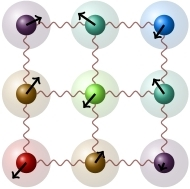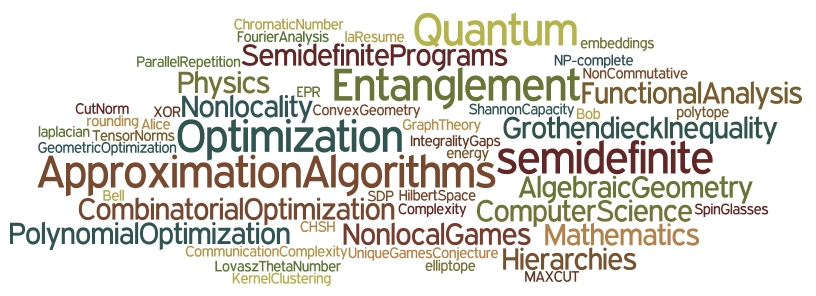Approximation Algorithms Quantum Information & Semidefinite Optimization

Abstract

This research project is about exploring the limits of efficient computation within classical and quantum computing, using semidefinite optimization as a main unifying tool.

Many fundamental problems in real-life applications and in scientific areas including physics, information processing and operations research, still lack a good computational solution. In fact most of them probably cannot be solved optimally with the current computing tools. This project aims to address this difficulty by combining two complementary routes: search for fast approximate solutions instead of optimal ones, and use quantum computing devices instead of classical ones. Our objective is to push the limits of efficient approximability and to understand the advantage of quantum effects in computations, now that large-scale quantum computers are getting closer to realization. The key tool that unifies this project is semidefinite optimization, a far-reaching generalization of the widely used techniques of linear programming, which in recent years is increasingly being used both in algorithm design and in quantum information.

This project is a unique cooperative effort of leading experts in approximation algorithms (Nikhil Bansal in the Department of Mathematics and Computer Science at Eindhoven University of Technology), quantum information (Harry Buhrman and Ronald de Wolf in the group Algorithms and Complexity), and semidefinite optimization (Monique Laurent in the group Networks and Optimization).

KeywordsApproximation Algorithms

A powerful, general method for designing approximation algorithms for discrete problems is to first solve a continuous relaxation of the problem, and then round the fractional solution to an integral solution for the discrete problem. Since the beginning of combinatorial optimization in the 50's, the most common aproach uses linear programming (LP) relaxations. Much progress has been made for constructing stronger LP relaxations and new rounding techniques. However, LPs have limited expressive power and are too weak for many problems.

Using semidefinite programming (SDP) instead of LPs has led to surprisingly strong results, including exact polynomial time algorithms for coloring perfect graphs, tractable bounds for the Shannon capacity of graphs, and the best known approximations for max-cut, graph partitioning, and discrepancy minimization. Moreover, SDP seems to capture the inherent computational hardness of many problems. For instance, assuming the Unique Game Conjecture (UGC), it is not possible to improve the known 0.878-approximation for max-cut!

Another reason for the growing interest in SDP for algorithm design is the emergence of the Lasserre hierarchy. This hierarchy has natural algebraic and functional-analytic interpretations, in terms of sums of squares of polynomials and the dual theory of moments, and it extends to optimization problems in non-commutative variables that arise naturally in the quantum setting. The Lasserre hierarchy constructs in a systematic way tighter SDP relaxations over a sequence of rounds, and produces after n (the number of variables) rounds an exponential-size program representing the original discrete program. Relaxations obtained after a constant number of rounds, however, are solvable in polynomial time and have led in recent years to striking progress on many fundamental questions such as unique games and sparsest cut.

Our broad goal is to develop new SDP based algorithmic tools, explore SDP based approaches for problems where only LP has been used so far, develop analogues of LP rounding techniques for SDP, and understand the limitations of the Lasserre hierarchy.

Quantum Information

Quantum mechanics is currently the most accurate theoretical model of Nature on atomic scales. Quantum information theory is a revolutionary new field of science and technology, whose central goal is to understand the power of quantum-mechanical systems for information processing. The field has been growing explosively since the 1990s, when it was first realized that quantum devices can be used to perform a number of information-processing tasks thought to be hard, or even impossible, in a classical world. For example, a quantum computer is able to factor an integer into its prime factors exponentially faster than any known classical algorithm.

Quantum systems can behave very differently from classical ones. In particular, two spatially separated quantum systems can be entangled, meaning that they are correlated in a non-classical way. Sharing entanglement allows remote parties to realize nonlocal correlations that are impossible to obtain classically, without imparting them with the ability to communicate instantaneously. This distinction is one of the most peculiar aspects of quantum theory and required many years to be properly appreciated.

Two broad objectives are to develop our understanding of entanglement and to study settings in which entanglement can be utilized as a tool in real-world situations. Entanglement can be studied quantitatively through the use of nonlocal games. Nonlocal games form a theoretical framework modelling physical experiments with which properties of entanglement can be tested in a lab. Recently, quantum variants of well-known graph parameters such as the chromatic number and the Shannon capacity were studied in the contexts of contrasting quantum mechanics with its classical variant, entanglement-assisted communication over noisy channels and optimization.

Nonlocal games provide a quantitative framework in which to study quantum entanglement and nonlocal quantum correlations. Consider the following game, played by Alice and Bob (who are on the same team) against Charlie. The game proceeds as follows: Charlie, whose strategy is fixed, sends Alice a bit a and Bob a bit b, choosing each bit independently and uniformly at random. Alice and Bob can agree on a strategy before the game begins but are not allowed to communicate once they receive questions. Alice and Bob win the game if they output one bit each, in such a way that the XOR of the bits they output is equal to a^b (the AND of the two inputs). In other words, they should output the same bit, except when both input bits are 1. A moment's reflection shows that their best strategy is to always output, say, 0. This allows them to win on three of the four possible inputs. It is also not difficult to show that equipping them with a shared source of randomness cannot help: the average success probability over the four possible questions will always be at most 3/4. Thus the maximum probability with which Alice and Bob can win, termed the classical value of the game, is 3/4. This game is the simplest example of a nonlocal game, and is also called XOR-game because the correlations between Alice and Bob are in the XOR of their outputs. What is remarkable is that if Alice and Bob are allowed to share quantum entanglement, then they can win the game with probability about .85, no matter which questions are asked. The maximum probability with which Alice and Bob can win if they share entanglement is termed the quantum value of the game.

Semidefinite optimization

Optimization typically involves searching for an optimal element in a huge complex structure of possible choices. A famous example of a combinatorial optimization problem is the max-cut problem, where one has to partition the vertices of a graph to maximize the number of the edges crossing the partition. Max-cut belongs to the general class of polynomial optimization problems, where the objective is to minimize or maximize a real-valued polynomial over a set of vectors that satisfy a number polynomial constraints. Other examples of polynomial optimization problems are finding optimal classical strategies for a given nonlocal game, determining the chromatic number of a graph and computing the cut-norm of a matrix. One can also consider polynomial optimization over non-commutative variables, which is more suitable for studying problems such as finding optimal quantum strategies for nonlocal games.

To turn a hard polynomial optimization problem into a tractable problem, one can relax it by modifying or dropping some of the constraints. By doing so, some of these problems can be turned into polynomial-time solvable semidefinite programs, whose optimum can be used to approximate the true optimum. A celebrated example of this is Goemans and Williamson's semidefinite programming relaxation of the max-cut problem. Certain nonlocal games known as XOR games can be relaxed similarly to get semidefinite programs that approximate the classical value (in fact, max-cut can be reduced to computing the classical value of an XOR game). A celebrated and fundamental result from functional analysis, Grothendieck's inequality, then shows that the classical value of XOR games can be approximated to within a factor 1.78... (this factor is known as Grothendieck's constant). As for the quantum value of XOR game, a surprising result of Tsirelson shows that these values are exactly the optimum of the semidefinite relaxation for the classical value!

Hierarchies of semidefinite programing relaxations involving increasing numbers of constraints, can be used to approximate optimization problems for which no good polynomial-time approxiamtion algortihms are known. Recently, hierarchies for polynomial optimization problems in non-commutative variables were discovered. These can be used, for example, to approximate the quantum value of nonlocal games and quantum analogues of classical graph parameters.

People

The following people are involved in research related to this project: Nikhil Bansal, Jop Briët, Harry Buhrman, Daniel Dadush, Dion Gijswijt, Monique Laurent, Teresa Piovesan, Giannicola Scarpa, Christian Schaffner, Florian Speelman, Frank Vallentin, Antonios Varvitsiotis, Ronald de Wolf

Open positions

PhD and postdoc positions are currently available for highly motivated people with a strong background in computer science and/or mathematics. You can apply by contacting Monique Laurent and/or sending your application to  nwosdpproject@gmail.com.

References

Consequences and Limits of Nonlocal Strategies (Richard Cleve, Peter Høyer, Ben Toner and John Watrous)

Non-locality and Communication Complexity (Harry Buhrman, Richard Cleve, Serge Massar and Ronald de Wolf)

Improved approximation algorithms for maximum cut and satisfiability problems using semidefinite programming (Michel Goemans and David Williamson)

Semidefinite Programming and Integer Programming (Monique Laurent and Franz Rendl)

Sums of squares, moment matrices and optimization over polynomials (Monique Laurent)

Convergent relaxations of polynomial optimization problems with non-commuting variables (Stefano Pironio, Miguel Navascues and Antonio Acin)

Trace-positive Polynomials, Sums of Hermitian Squares and the Tracial Moment Problem (Sabine Burgdorf)

Constructive algorithms for discrepancy minimization (Nikhil Bansal)

Min-max graph partitioning and small set expansion (Nikhil Bansal, Uriel Feige, Robi Krauthgamer, Konstantin Makarychev, Viswanath Nagarajan, Joseph Naor and Roy Schwartz)

Grothendieck Inequalities, Nonlocal Games and Optimization (Jop Briët)

Grothendieck-type inequalities in combinatorial optimization (Subash Khot and Assaf Naor)

Linear vs. semidefinite extended formulations: exponential separation and strong lower bounds (Samuel Fiorini, Serge Massar, Sebastian Pokutta, Hans Raj Tiwary, and Ronald de Wolf)

Quantum Entanglement in Non-local Games, Graph Parameters and Zero-error Information Theory (Giannicola Scarpa)

Zero-error source-channel coding with entanglement (Jop Briet, Harry Buhrman, Monique Laurent, Teresa Piovesan and Gianni Scarpa)

Combinatorial Conditions for Low Rank Solutions in Semidefinite Programming (Antonios Varvitsiotis)